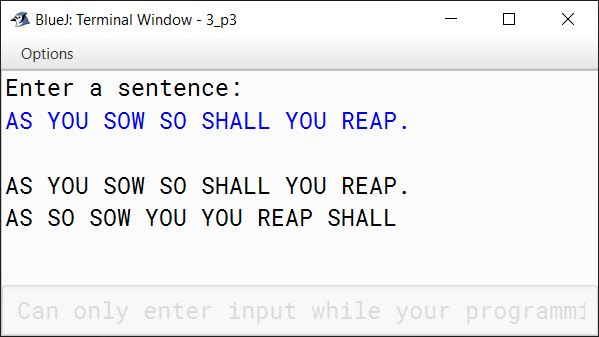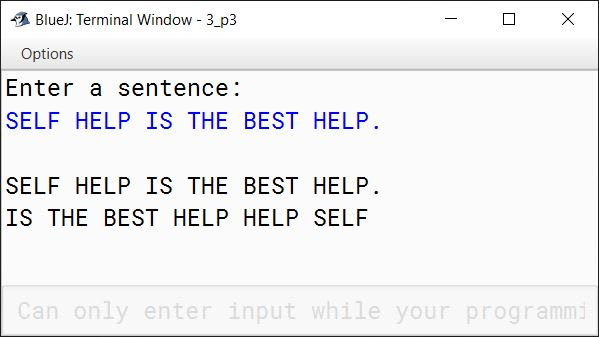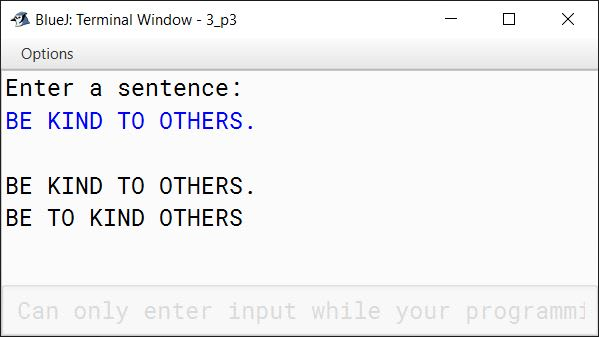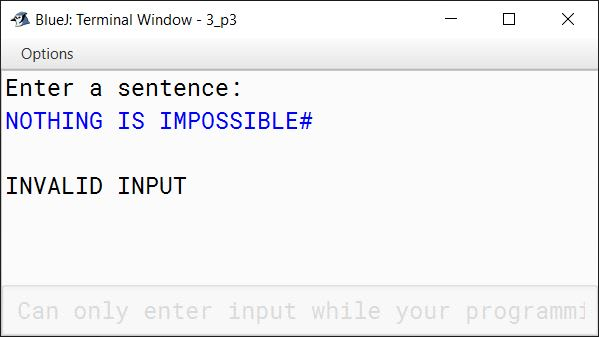# Solved 2020 Practical Paper ISC Computer Science

## Practical Questions

#### Question 1

A Prime-Adam integer is a positive integer (without leading zeros) which is a prime as well as an Adam number.

Prime number: A number which has only two factors, i.e. 1 and the number itself.

Example: 2, 3, 5, 7 ... etc.

Adam number: The square of a number and the square of its reverse are reverse to each other.

Example: If n = 13 and reverse of 'n' = 31, then,

(13)2 = 169

(31)2 = 961 which is reverse of 169

thus 13, is an Adam number.

Accept two positive integers m and n, where m is less than n as user input. Display all Prime-Adam integers that are in the range between m and n (both inclusive) and output them along with the frequency, in the format given below:

Test your program with the following data and some random data:

Example 1

INPUT:
m = 5
n = 100

OUTPUT:
11 13 31
FREQUENCY OF PRIME-ADAM INTEGERS IS: 3

Example 2

INPUT:
m = 100
n = 200

OUTPUT:
101 103 113
FREQUENCY OF PRIME-ADAM INTEGERS IS: 3

Example 3

INPUT:
m = 50
n = 70

OUTPUT:
NIL
FREQUENCY OF PRIME-ADAM INTEGERS IS: 0

Example 4

INPUT:
m = 700
n = 450

OUTPUT:
INVALID INPUT

##### Solution
```import java.util.Scanner;

{
public static int reverse(int num) {
int rev = 0;

while (num != 0) {
int d = num % 10;
rev = rev * 10 + d;
num /= 10;
}

return rev;
}

public static boolean isAdam(int num) {
int sqNum = num * num;
int revNum = reverse(num);
int sqRevNum = revNum * revNum;
int rev = reverse(sqNum);

return rev == sqRevNum;
}

public static boolean isPrime(int num) {
int c = 0;

for (int i = 1; i <= num; i++) {
if (num % i == 0) {
c++;
}
}

return c == 2;
}

public static void main(String args[]) {
Scanner in = new Scanner(System.in);
System.out.print("Enter the value of m: ");
int m = in.nextInt();
System.out.print("Enter the value of n: ");
int n = in.nextInt();
int count = 0;

if (m >= n) {
System.out.println("INVALID INPUT");
return;
}

for (int i = m; i <= n; i++) {
boolean prime = isPrime(i);
if (prime) {
System.out.print(i + " ");
count++;
}
}
}

if (count == 0) {
System.out.print("NIL");
}

System.out.println();
System.out.println("FREQUENCY OF PRIME-ADAM INTEGERS IS: " + count);
}
}```
##### Output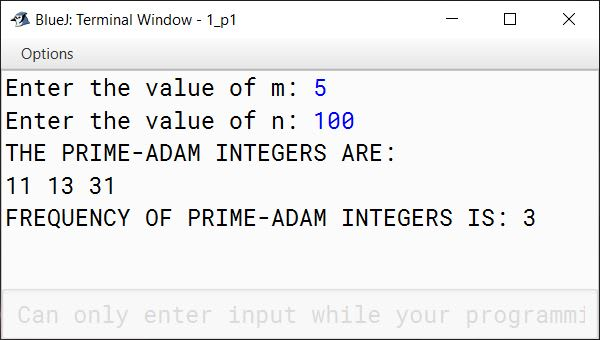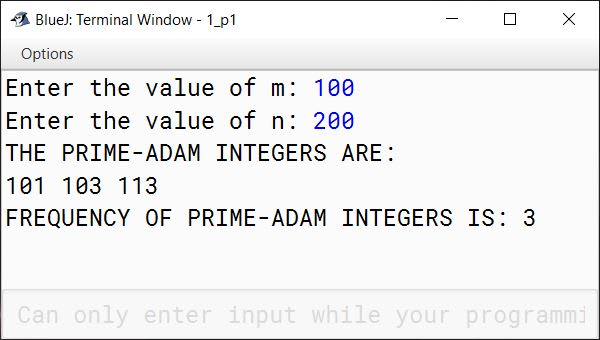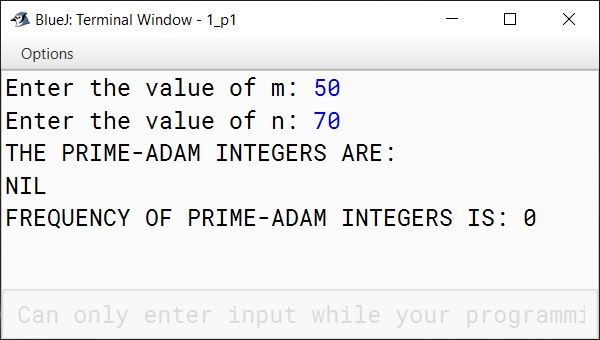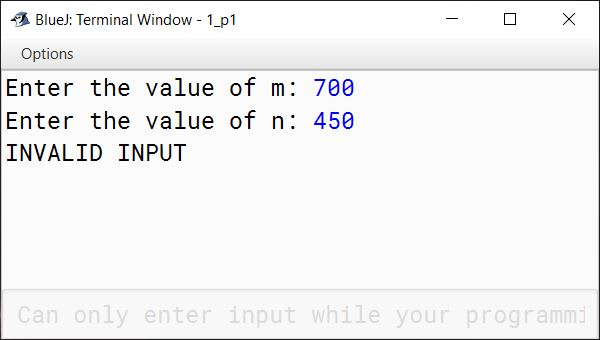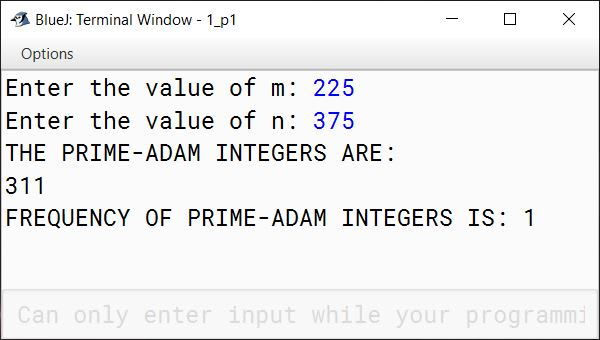#### Question 2

Write a program to declare a matrix A[][] of order (M x N) where 'M' is the number of rows and 'N' is the number of columns such that the value of 'M' must be greater than 0 and less than 10 and the value of 'N' must be greater than 2 and less than 6. Allow the user to input digits (0 - 7) only at each location, such that each row represents an octal number.

Example:

 2 3 1 (decimal equivalent of 1st row = 153 i.e. 2x82 + 3x81 + 1x80) 4 0 5 (decimal equivalent of 2nd row = 261 i.e. 4x82 + 0x81 + 5x80) 1 5 6 (decimal equivalent of 3rd row = 110 i.e. 1x82 + 5x81 + 6x80)

Perform the following tasks on the matrix:

1. Display the original matrix.
2. Calculate the decimal equivalent for each row and display as per the format given below.

Test your program for the following data and some random data:

Example 1:

INPUT:
M = 1
N = 3
ENTER ELEMENTS FOR ROW 1: 1 4 4

OUTPUT:

FILLED MATRIXDECIMAL EQUIVALENT
144100

Example 2:

INPUT:
M = 3
N = 4
ENTER ELEMENTS FOR ROW 1: 1 1 3 7
ENTER ELEMENTS FOR ROW 2: 2 1 0 6
ENTER ELEMENTS FOR ROW 3: 0 2 4 5

OUTPUT:

FILLED MATRIXDECIMAL EQUIVALENT
1137607
21061094
0245165

Example 3:

INPUT:
M = 3
N = 3
ENTER ELEMENTS FOR ROW 1: 2 4 8

OUTPUT:
INVALID INPUT

Example 4:

INPUT:
M = 4
N = 6

OUTPUT:
OUT OF RANGE

##### Solution
```import java.util.Scanner;

public class OctalMatrix
{
public static void main(String args[]) {
Scanner in = new Scanner(System.in);
System.out.print("Enter the number of rows (M): ");
int m = in.nextInt();
System.out.print("Enter the number of columns (N): ");
int n = in.nextInt();

if (m <= 0 || m >= 10 || n <= 2 || n >= 6) {
System.out.println("OUT OF RANGE");
return;
}

int a[][] = new int[m][n];

for (int i = 0; i < m; i++) {
System.out.println("ENTER ELEMENTS FOR ROW " + (i + 1) + ": ");
for (int j = 0; j < n; j++) {
a[i][j] = in.nextInt();
if (a[i][j] < 0 || a[i][j] > 7) {
System.out.println("INVALID INPUT");
return;
}
}
}

System.out.println("FILLED MATRIX\tDECIMAL EQUIVALENT");

for (int i = 0; i < m; i++) {
int decNum = 0;
for (int j = 0; j < n; j++) {
decNum += a[i][j] * Math.pow(8, n - j - 1 );
System.out.print(a[i][j] + " ");
}
System.out.print("\t\t" + decNum);
System.out.println();
}
}
}```
##### Output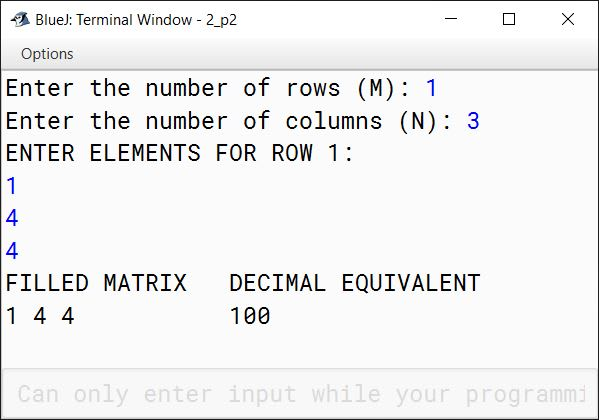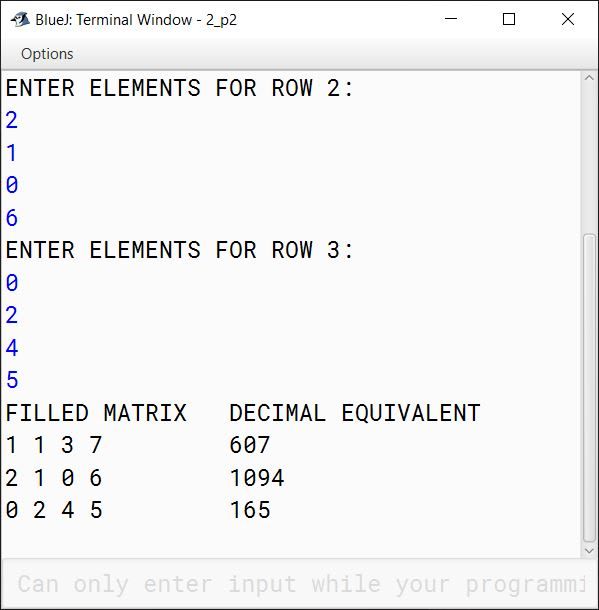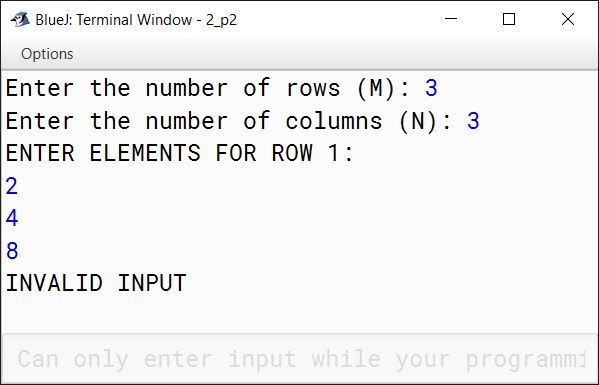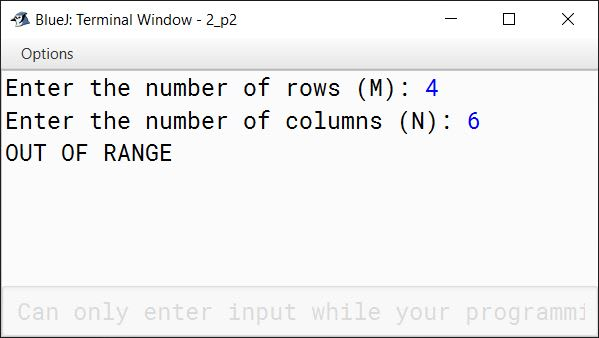#### Question 3

Write a program to accept a sentence which may be terminated by either '.', '?' or '!' only. The words are to be separated by a single blank space and are in UPPER CASE.

1. Check for the validity of the accepted sentence only for the terminating character.
2. Arrange the words in ascending order of their length. If two or more words have the same length, then sort them alphabetically.
3. Display the original sentence along with the converted sentence.

Test your program for the following data and some random data:

Example 1:

INPUT:
AS YOU SOW SO SHALL YOU REAP.

OUTPUT:
AS YOU SOW SO SHALL YOU REAP.
AS SO SOW YOU YOU REAP SHALL

Example 2:

INPUT:
SELF HELP IS THE BEST HELP.

OUTPUT:
SELF HELP IS THE BEST HELP.
IS THE BEST HELP HELP SELF

Example 3:

INPUT:
BE KIND TO OTHERS.

OUTPUT:
BE KIND TO OTHERS.
BE TO KIND OTHERS

Example 4:

INPUT:
NOTHING IS IMPOSSIBLE#

OUTPUT:
INVALID INPUT

##### Solution
```import java.util.*;

public class StringCheck
{
public static String sortString(String ipStr) {
StringTokenizer st = new StringTokenizer(ipStr);
int wordCount = st.countTokens();
String strArr[] = new String[wordCount];

for (int i = 0; i < wordCount; i++) {
strArr[i] = st.nextToken();
}

for (int i = 0; i < wordCount - 1; i++) {
for (int j = 0; j < wordCount - i - 1; j++) {
if (strArr[j].length() > strArr[j + 1].length()) {
String t = strArr[j];
strArr[j] = strArr[j+1];
strArr[j+1] = t;
}

if (strArr[j].length() == strArr[j + 1].length()
&&(strArr[j].compareTo(strArr[j+1]) > 0))
{
String t = strArr[j];
strArr[j] = strArr[j+1];
strArr[j+1] = t;
}
}
}

StringBuffer sb = new StringBuffer();
for (int i = 0; i < wordCount; i++) {
sb.append(strArr[i]);
sb.append(" ");
}

/*
* trim will remove the extra space added
* to the end of the string in the loop above
*/
return sb.toString().trim();
}

public static void main(String args[]) {
Scanner in = new Scanner(System.in);
System.out.println("Enter a sentence:");
String str = in.nextLine();
int len = str.length();
System.out.println();

if (str.charAt(len - 1) != '.'
&& str.charAt(len - 1) != '?'
&& str.charAt(len - 1) != '!') {
System.out.println("INVALID INPUT");
return;
}

/*
* str.substring(0, len - 1) removes the
* '.', '?', '!' at the end of the string
*/
String sortedStr = sortString(str.substring(0, len - 1));

System.out.println(str);
System.out.println(sortedStr);
}
}```
##### Output